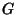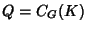## Aschbacher's Component Theorem

Suppose that(the commuting product of all components of) is Simple andcontains a Semisimple Involution. Then there is some Semisimple Involutionsuch thathas a Normal Subgroupwhich is either Quasisimple or Isomorphic toand such thatis Tightly Embedded.

See also Involution (Group), Isomorphic Groups, Normal Subgroup, Quasisimple Group, Simple Group, Tightly Embedded